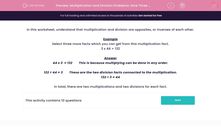# Multiplication and Division Problems: Give Three Facts

In this worksheets, students select facts connected to the given multiplication/division statement.This content is premium and exclusive to EdPlace subscribers.Key stage:  KS 2

Curriculum topic:   Maths and Numerical Reasoning

Curriculum subtopic:   Word Problems

Difficulty level:#### Worksheet Overview

In this worksheet, understand that multiplication and division are opposites, or inverses of each other.

Example

Select three more facts which you can get from this multiplication fact.

3 x 44 = 132

44 x 3  = 132        This is because multiplying can be done in any order.

132 ÷ 44 = 3        These are the two division facts connected to the multiplication.

132 ÷ 3 = 44

In total, there are two multiplications and two divisions for each fact.

### What is EdPlace?

We're your National Curriculum aligned online education content provider helping each child succeed in English, maths and science from year 1 to GCSE. With an EdPlace account you’ll be able to track and measure progress, helping each child achieve their best. We build confidence and attainment by personalising each child’s learning at a level that suits them.

Get started